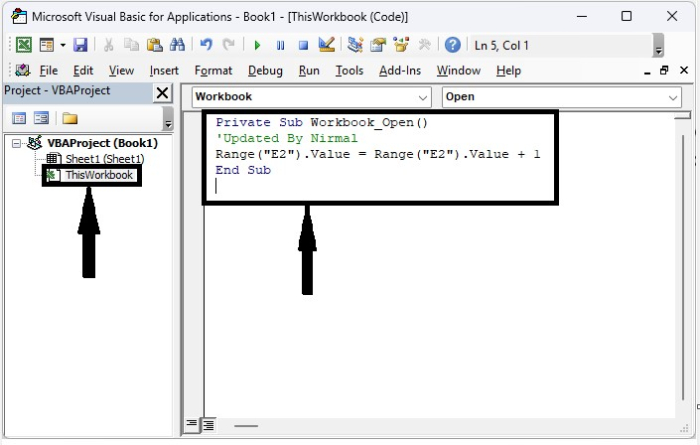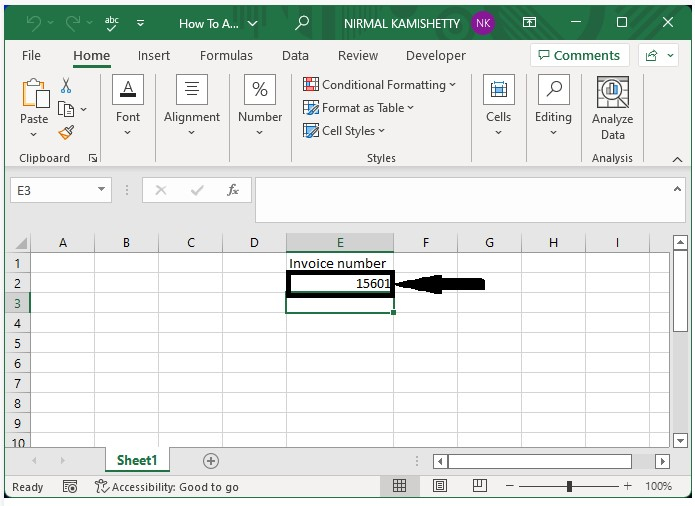# How to Automatically Generate the Invoice Numbers in Excel?

An invoice number is a number that is used as a unique identifier for any business in order to keep track of their records. The main requirement for invoice numbers is that they are distinct from one another and that they are in sequential order. If we try to use the numbers with the auto-fill handle, there is a chance that the numbers could have been duplicated or missed, but we can apply the special process to reduce those errors. This tutorial will help you understand how we can automatically generate invoice numbers in Excel.

## Automatically Generate Invoice Number in Excel

Here we will apply the VBA code for the whole workbook. Let us see an uncomplicated process to see how we can automatically generate invoice numbers in Excel. We need to use the help of a VBA application, as it can’t be completed directly in Excel.

Step 1

Let us consider a new Excel workbook and then type the first invoice number. In our case, type 15600 in cell E2. The invoice number will start at 15600.

Then, right-click on the sheet name and choose View Code to open the VB application, then double-click on this workbook, and finally, enter the programme into the text box as shown in the image below. The code will apply to the whole workbook.

### Example

Private Sub Workbook_Open()
‘Updated By Nirmal
Range("E2").Value = Range("E2").Value + 1
End Sub


In the program, cell E2 is the one where we have entered our number.Step 2

Save the sheet as a macro-enabled template and close the workbook. Now, whenever we close and open the workbook, the number in cell E2 will be increased by one, as shown in the image below. The code won’t work if we save the workbook as a regular sheet.## Conclusion

In this tutorial, we used a simple example to demonstrate how we can automatically generate invoice numbers in Excel.